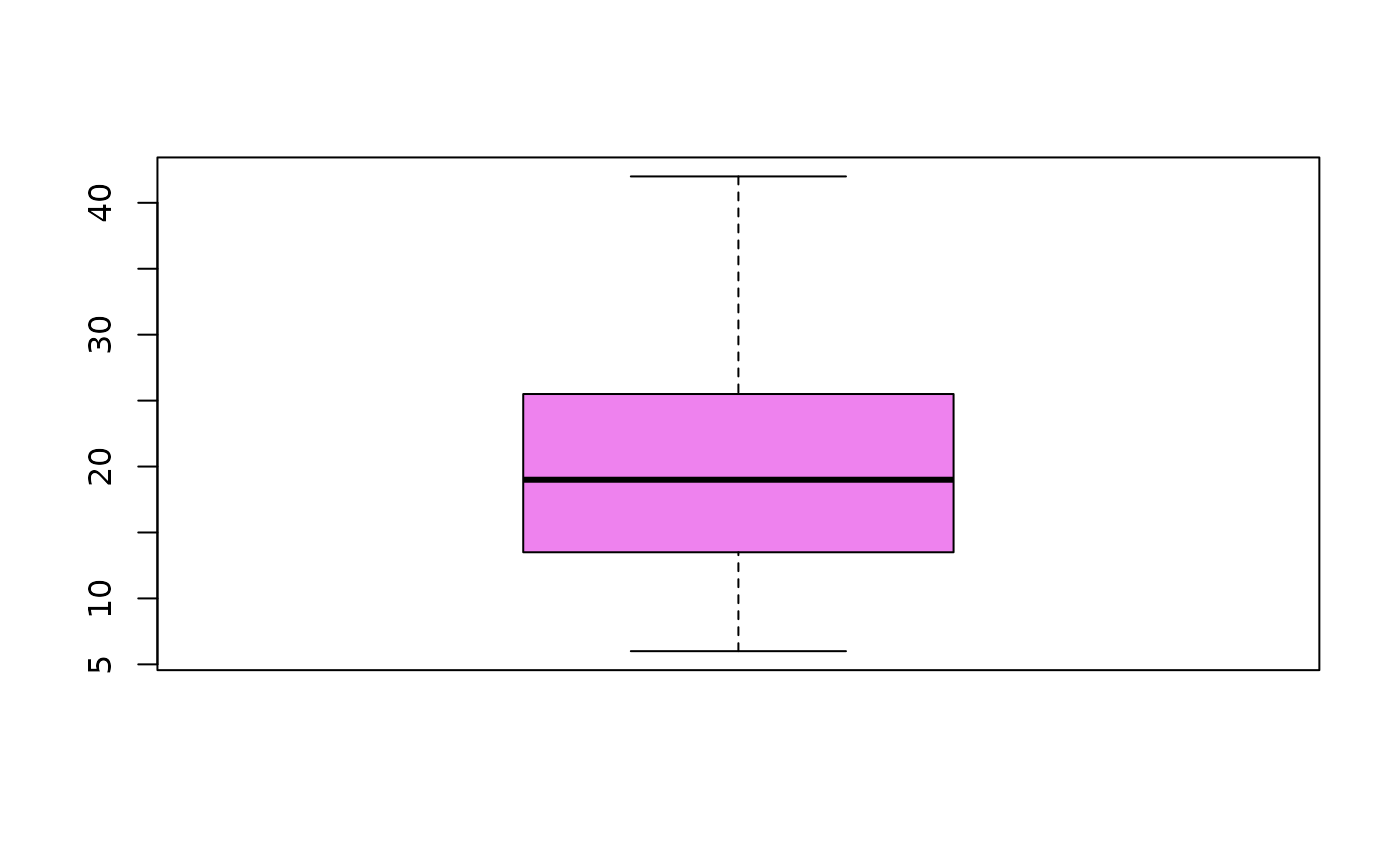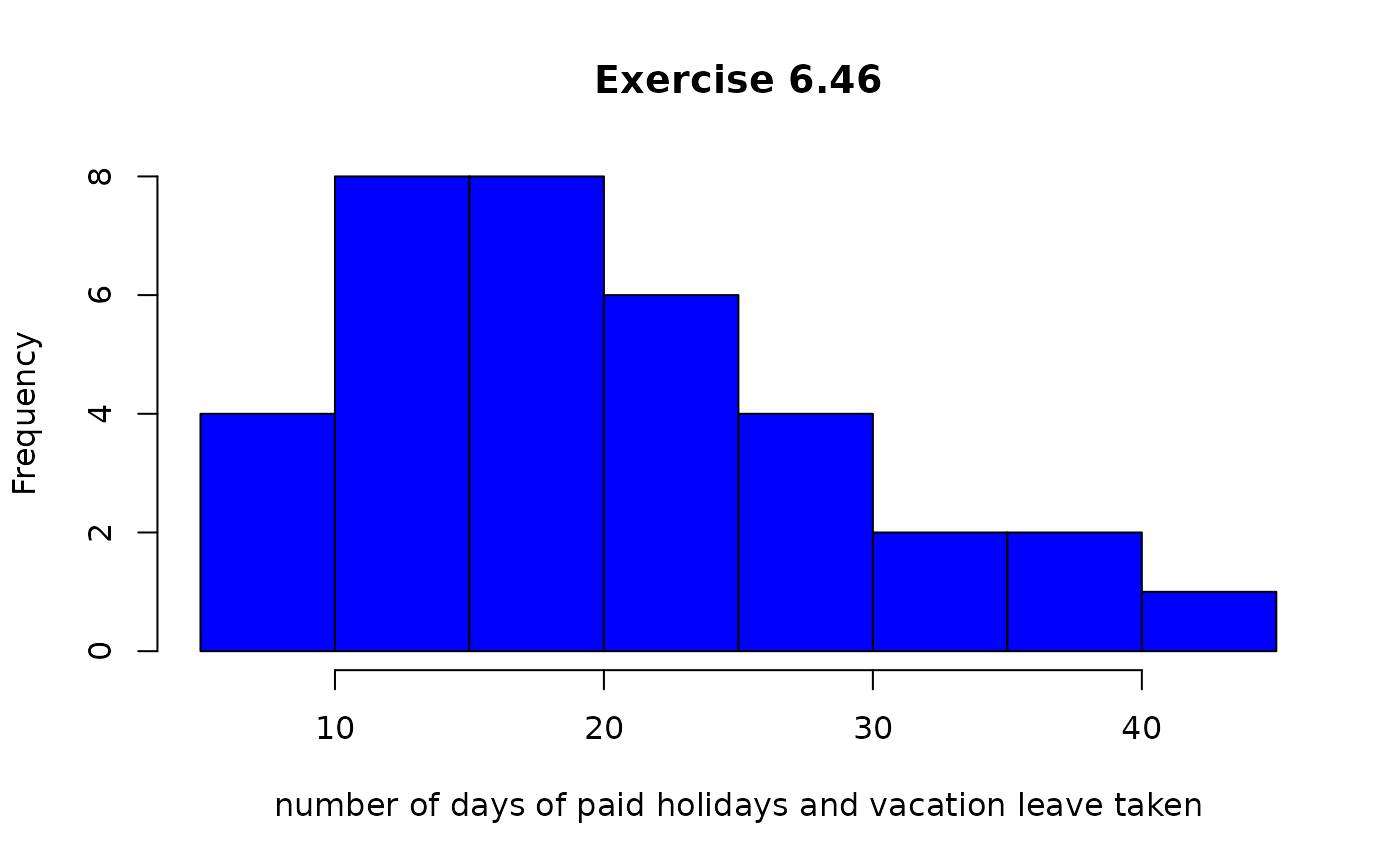Data for Exercise 6.46 and 6.98

Vacation

## Format

A data frame/tibble with 35 observations on one variable

number

number of days of paid holidays and vacation leave taken

## Examples


boxplot(Vacation$number, col = "violet")hist(Vacation$number, main = "Exercise 6.46", col = "blue",
xlab = "number of days of paid holidays and vacation leave taken")t.test(Vacation$number, mu = 24) #> #> One Sample t-test #> #> data: Vacation$number
#> t = -2.2584, df = 34, p-value = 0.03045
#> alternative hypothesis: true mean is not equal to 24
#> 95 percent confidence interval:
#>  17.37768 23.65089
#> sample estimates:
#> mean of x
#>  20.51429
#>